# Further Application — The Brachistochrone Problem

The curve of fastest descent:  Drop a point object on a curved slope — what slope gives the minimum time for it to travel between two points?

This problem is different from the others:

1. Unlike the beam and chain, both of which need to have the same length after bending, we don’t care how long the slope is — only that it gives the minimum time.

2. Angles don’t seem to enter into it much.  They don’t enter directly (as hinges in the beam problem) or even necessarily as natural variables (as in the catenary problem) (since length and angles are naturally conjugate).

However, it is a similar problem in that

1. We’re trying to find an extreme of a function

2. We can do approach the problem by breaking up the curve into a bunch of parts, each parametrized by a variable, and then (in principle, at least) solving for the variables.

Although this is not exactly the usual way of doing it, what I like about this approach is

-Problems set up this way tend to lend themselves to numerical solutions, if necessary.

-It requires a relative minimum of ingenuity — if you set up the problem right, the answer tends to pop out.

(This is not exactly true for this case — I may hint at how things could go “wrong”)

This is how we set up the problem:We divide the path into a bunch of line segments, with x dimension widths dx and y dimension widths dy.  Cartesian coordinates seem to make sense for this problem, since the y coordinate is “special” (potential energy depends only on y).

We can assume the lines are short enough that the particle has a fixed speed as it travels across one, only changing speeds when it comes to a new line.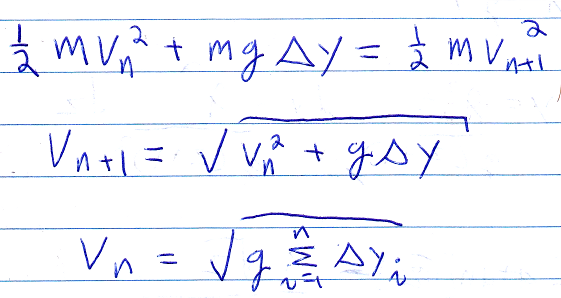The first equation above is just the conservation of energy.  The second uses the first to solve for the velocity for the next line.  Then the final equation is the total velocity after n line segments, assuming that the particle is dropped (starts with v=0).

The length of each segment is sqr(dx^2+dy^2), so the time to cross each line is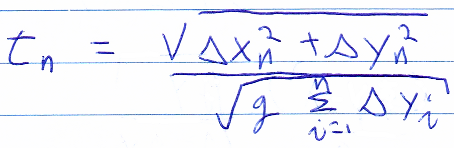Then we want to find the minimum time (the sum of all those tn’s), subject to the curve going through the point (a,b).  SoI said that for this problem, the right answer doesn’t necessarily pop right out, and one way we could make this problem much harder is by considering the gradient with respect to the dy’s.  They are all coupled, so solving for them would be very difficult.  Instead, we will just use the gradient with respect to the dx’s.

We could think of this in two ways:

-Doing it with respect to the dx’s is enough to solve the problem, so who cares about the other Lagrangian multiplier.

-We could assume the dy’s are “fixed”, in that we divide the vertical path into equally spaced segments and only care about solving for the dx’s as a function of y.  We probably wouldn’t know to do this ahead of time (why not consider dx fixed and solve for dy?), but we left the problem open enough to take that path now.  Another paradoxical issue is that, and yes we are slightly cheating here by anticipating the answer in even considering this now, depending on the exact location of the finishing point relative to the beginning, the particle may first go down, but then up.  So dy is not necessary b/n, even for fixed, even divisions of y.  Nevertheless, this works.(in case my writing is unclear, both square roots are in the denominator)

We can solve for dx: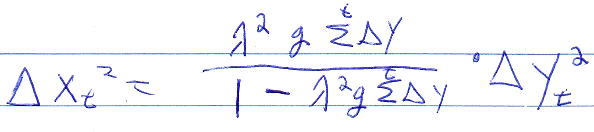I guess we didn’t really need to square both sides of the equation, because we can now take the square root, let n go to infinity, and integrate.  delta-x and -y really become dx and dy then, and the sum of the delta-y’s becomes simply y.  So we haveOddly, the TI89 cannot do that integral on its own:But we can make a fairly obvious substitution manually: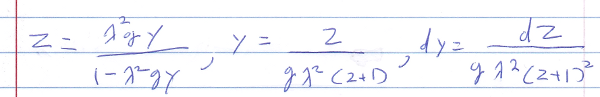And then the TI89 can do it:I guess this gives me an opportunity to go on an off-topic rant.  Some people who like old school, purely numeric calculators (eg, hp15c, hp71b, hp42, Sharp PC-1500) think CAS calculators are dumb because you only would need them in school, or they do all your work for you (which seem to be mutually exclusive complaints, but hey).

I don’t think either complaint is true.  The first seems to suggest that they can only do simple things, like integrate sin(x).  But, no, they can handle fairly complex expressions, and they might be better at it than a computer (ie., Mathematica) for the same reason any calculator can be preferred to using a computer (even for number crunching): They are so much easier to use. Formula entry is particularly easier with a calculator keyboard. (Entering formulas like that is roughly infinitely percent easier and faster than doing it in Microsoft Equation Editor or LaTeX; it’s roughly comparable to writing them down by hand on paper).

Granted, there are some things Mathematica can do that a CAS calculator cannot (eg., express non-elementary integrals in terms of a hypergeometric function), but even then a CAS calculator can still give you a leg up versus doing the problem completely on paper.

The second complaint I think is disproved here: A little bit of prodding or knowledge of how you’d do the problem manually or even creativity makes these calculators more useful.

Basically, I think CAS calculators do exactly what any calculator should do:  Get rid of the tedium so you can focus on the more fun aspects of math.

</rant>

We could take an integration constant, but, spoiler alert, it would have to equal zero anyway for the curve to go through (0,0).

Another thing we could note is that every time lambda appears, it’s squared and next to g.  Since lambda remains to be solved for, and we don’t really care about g (changing g just changes whatever lambda is) we can replace lambda ^2-g with another variable, say p.

(We might care about g if we didn’t want to just find the curve, but find the location along the curve as a function of time in seconds)

So the solution is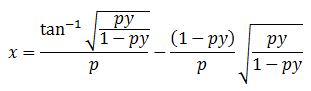We might as well say at this point that this curve is a cycloid.

p is used a parameter to ensure the curve goes through (a,b).  If the particle goes down then up, we will need to use the second half of the cycloid, which we get by replacing all square roots with their negatives, but also taking the second branch of atan (in other words, by adding pi), getting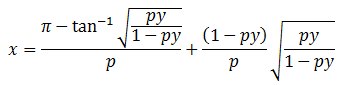In either case, I think p would have to be solved numerically.

I made an Excel file to do that.  Solving for p is somewhat tricky, in that using Newton’s method can result in a sequence that spirals out of control.  So instead of simply using

x – f(x)/f'(x)

I made it possible to use

x- s f(x)/f'(x)

If s (which in the spreadsheet is in I6) is less than 1, it should still converge, just more slowly / conservatively.

As is, the solution seems to be fairly robust, regardless of which half of the cycloid the end point is on.

The spreadsheet also, for the heck of it, includes a graph that shows both the cycloid and a circle that goes through the two points, and starts with a pure vertical drop. It seems the reason the circular path takes more time is that is longer, even if there’s a higher maximum speed.

I was thinking of making another spreadsheet to animated a two particle “race” down different curves, but I think I spent too much time on this post already.